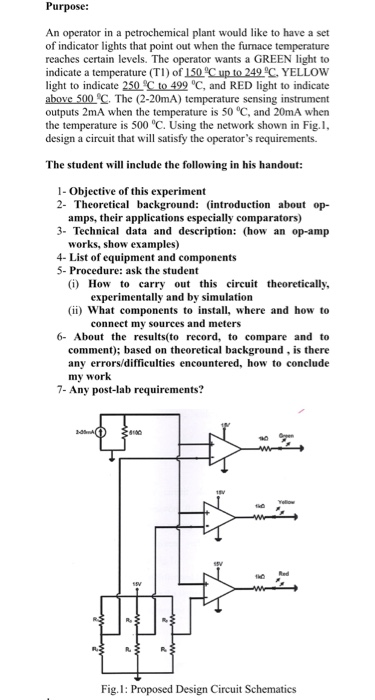# Purpose: An operator in a petrochemical plant would like to have a set of indicator lights that point out when

###### Question:Purpose: An operator in a petrochemical plant would like to have a set of indicator lights that point out when the furnace temperature reaches certain levels. The operator wants a GREEN light to indicate a temperature (TI) of 150 C up to 249 C YELLOW light to indicate 250 C to 499 °C, and RED light to indicate above 500 SC. The (2-20mA) temperature sensing instrument outputs 2mA when the temperature is 50 °C, and 20mA when the temperature is 500 °C. Using the network shown in Fig.I design a circuit that will satisfy the operator's requirements. The student will include the following in his handout: 1- Objective of this experiment 2- Theoretical background: introduction about op- amps, their applications especially comparators) 3- Technical data and description: (how an op-amp works, show examples) 4- List of equipment and components 5- Procedure: ask the student (i) How to crry out this circuit theoretically, experimentally and by simulation (ii) what components to install, where and how to connect my sources and meters 6-About the results(to record, to compare and to comment); based on theoretical background, is there any errors/difficulties encountered, how to conclude my work 7- Any post-lab requirements? Fig.I: Proposed Design Circuit Schematics

#### Similar Solved Questions

##### Let U be a continuous random variable with the following probability density function: g(t) = 1+t...
Let U be a continuous random variable with the following probability density function: g(t) = 1+t -1<t< 0 1-t 0<t<1 0 otherwise a. Verify that g(t) is indeed a probability density function.  b. Compute the expected value, E(U), and variance, V(U), of U. (10)...
##### How do you solve (16+ \frac { 878} { 26} ) ^ { 3t } = 2?
How do you solve (16+ \frac { 878} { 26} ) ^ { 3t } = 2?...